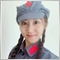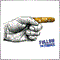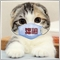# oncalculate函数问题！120

```int OnCalculate(const int rates_total,
const int prev_calculated,
const datetime &time[],
const double &open[],
const double &high[],
const double &low[],
const double &close[],
const long &tick_volume[],
const long &volume[],
{```

MQL参考文件中，罗列了Oncalculate函数的机制：

1、只在自定义指标中调用

2、每次价格变化，Oncalculate函数调用一次

3、可以，用不同的价格状态（如：开盘、收盘等）计算指标。

4、参数：

（1）rates_total（图标中，k线的总数量，含波动中的K线？）

（2）prev_calculated（图标中，k线的数量，不含波动中的K线？）

（3）各种数组（已存放的各种数据，计算指标时使用）

5、函数返回：prev_calculated

1、参数rates_total和prev_calculated应该是有区别的，但我的理解应该有误，因为在使用《参考文件》的案例时，rates_total和prev_calculated是相等的。

2、参考文件中的这两层意思完全没有懂，希望高手翻译并指导。

We should note the connection between the return value of OnCalculate() and the second input parameter prev_calculated. During the function call, the prev_calculated parameter contains a value returned by OnCalculate() during previous call. This allows for economical algorithms for calculating the custom indicator in order to avoid repeated calculations for those bars that haven't changed since the previous run of this function.

For this, it is usually enough to return the value of the rates_total parameter, which contains the number of bars in the current function call. If since the last call of OnCalculate() price data has changed (a deeper history downloaded or history blanks filled), the value of the input parameter prev_calculated will be set to zero by the terminal.

3、Oncalculate函数是否还有其他机制，请高手指导，谢谢。4112

helloea:

1、参数rates_total和prev_calculated应该是有区别的，但我的理解应该有误，因为在使用《参考文件》的案例时，rates_total和prev_calculated是相等的。

1、若第一次函数调用，prev_calculated=0，rates_total=10，函数结束退出后，prev_calculated=10，rates_total=10，这个应该能理解吧；

2、非第一次函数调用，如果没有生成新 K 线，那么，即使最后那根 K 线价格更新了若干次，prev_calculated 总是等于 rates_total，这也好理解吧；

3、非第一次函数调用，如果生成了新 K 线，此时函数进入时 prev_calculated=10，rates_total=11，而在函数结束退出后，prev_calculated=11，rates_total=11，这个不难理解吧；39